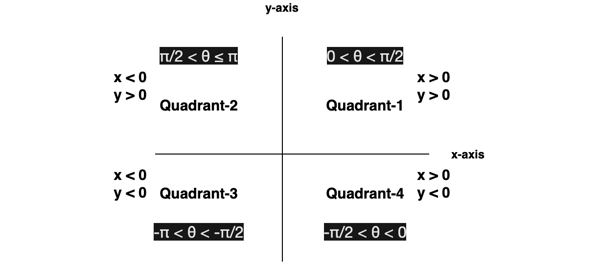Trusted answers to developer questions
Trusted Answers to Developer Questions

Related Tags

javascript
math
communitycreator

# What is Math.atan2() in JavaScript?Arslan Bajwa

Grokking Modern System Design Interview for Engineers & Managers

Ace your System Design Interview and take your career to the next level. Learn to handle the design of applications like Netflix, Quora, Facebook, Uber, and many more in a 45-min interview. Learn the RESHADED framework for architecting web-scale applications by determining requirements, constraints, and assumptions before diving into a step-by-step design process.

Math is a built-in object in JavaScript that contains different methods and properties used to perform mathematical operations. It contains the atan2() function which is used to compute the angle between the positive x-axis and the ray originating from (0,0) to (x,y) points.

## Syntax

Math.atan2(y,x);

## Parameter

• x: It is of type Number and indicates the x-coordinate of the input point.
• y: It is of type Number and indicates the y-coordinate of the input point.

Number in JavaScript is a 64-bit double-precision value that is the same as double in C# or Java.

## Return valueAngle: It returns the angle θ between the positive x-axis and the ray starting from point (0,0) to the point (x,y). It is measured in radians. Its range is -π <= θ <= π (radians)

π is a mathematical constant whose value is equal to 3.14159 approximately.

## Code

console.log("atan2(0,0) = " + Math.atan2(0,0));

console.log("atan2(-10,-10) = " + Math.atan2(-10,-10));

console.log("atan2(10,10) = " + Math.atan2(10,10));

console.log("atan2(10,-10) = " + Math.atan2(10,-10));

console.log("atan2(-10,10) = " + Math.atan2(-10,10));

console.log("atan2(∞,10) = " + Math.atan2(Infinity,10));

console.log("atan2(10,-∞) = " + Math.atan2(10,-Infinity));

RELATED TAGS

javascript
math
communitycreator

CONTRIBUTORArslan Bajwa

Grokking Modern System Design Interview for Engineers & Managers

Ace your System Design Interview and take your career to the next level. Learn to handle the design of applications like Netflix, Quora, Facebook, Uber, and many more in a 45-min interview. Learn the RESHADED framework for architecting web-scale applications by determining requirements, constraints, and assumptions before diving into a step-by-step design process.

Keep Exploring

Learn in-demand tech skills in half the time